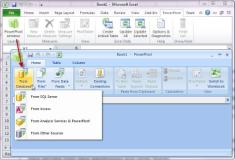# PowerPivots with Excel 2010 & 2013

PowerPivot Introduction Training in Belfast & Northern Ireland

PowerPivot is an add-in for Microsoft Excel 2010 that enables you to:

• Import millions of rows of data from multiple data sources into a single Excel workbook
• Create relationships between heterogeneous data
• Create calculated columns and measures using formulas
• Build PivotTables and PivotCharts, and then further analyse the data so that you can make timely business decisions without requiring IT assistance.

Our 2 Day PowerPivot training course will enable delegates to maximise the benefit of this useful tool.

Prerequisites

This course is designed for Advanced users - a knowledge of Pivot tables and Advanced functions is essential.Introduction

• What is PowerPivot?

• What is a PivotTable?

• Example with classical Excel PivotTable

• First Steps with PowerPivot
• Example with PowerPivot

Basic PowerPivot Concepts

• Formatting Numbers

• Handling Technical and Useless Columns

• Understanding Calculated Columns

• Using Lookup Tables

• Understanding Calculated Fields

• Handling Many Tables

• Refreshing Data

• Using Slicers

SharePoint Integration

• PowerPivot for SharePoint

• PowerPivot Gallery

• Publishing an Excel Workbook
• Parameters Pane

• PowerPivot Data Refresh
• Data Connections

• User Credentials

Power View

• Creating a Power View Report

• Inserting New Views in Power View

• Type of Charts & Maps

• Applying Filters to Power View Reports

• Creating Tiles

Shaping Reports

• Defining KPIs

• Creating Hierarchies

• Properties for Power View Reports

• Named Sets in Excel

• Using Perspectives

• Drillthrough with PowerPivot

• Understanding Data Connections

• Different Kind of Connections

• Using Existing Connections

• Filtering Options

• Issues in the Query Designer

• Best Practices

• The MDX Query Designer

• Handling of Keys in SSAS

• OLAP cube or DataMart?

• Using the Schema.INI configuration

• Using Copy & Paste Operations

• Reporting Services Reports

• Internet Data Feeds

• Windows Azure Data Market

Understanding Data Models

• What is a Data Model?

• Why PowerPivot Users need Data Modelling?

• Physical and Logical Data Models
• Normalization and Denormalization

• Empty and Default Values

• Understanding How and When to Denormalize

• SQL Query Designer as a Data Modelling Tool

• Different kinds of Joins

• Setting Relationship Manually

• Understanding OUTER Joins

Introduction to DAX

• Calculation Foundations

• DAX Data Types

• DAX Operators

• Calculated Columns Examples

• Calculated Fields Examples

• Introduction to the Execution Context (Row and Filter)

• Choosing Between Calculated Columns and Calculated Fields

• Handling Errors in DAX

• Common DAX Functions Examples

• Statistical and Logical Functions

• Information Functions

• Mathematical Functions

• Text Functions

• Date and Time Functions

Table Functions and CALCULATE

• Filtering Table with FILTER

• Mixing ALL and Other Filters

• Use of RELATEDTABLE

• CALCULATE Examples and Relationships

Time Intelligence in PowerPivot

• Why a Calendar Table is Useful

• Attribute Consolidation

• Common Calendar Calculations

• Creating a Calendar Table with Excel

• Excel Tips to Create a Calendar Table

• Working Days Calculation

• Common Calendar Calculations

• X To Date (YTD, QTD, MTD)

• Same Period Last Year

• Other Custom Aggregation Function

• Delta Over Previous Year

Basic DAX Patterns

• Banding in PowerPivot

• New and Returning Customers

• Compare Budget and Sales

• Many-to-Many Relationships

• Merge Values from Different Tables

• ABC / Pareto Analysis

PowerPivot Intro